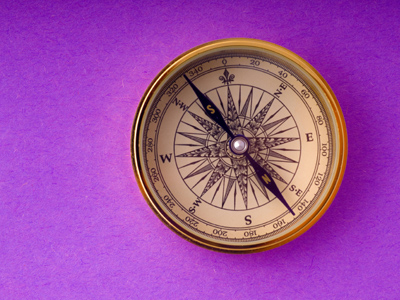The compass point opposite South East is North West.

# Position (Year 4)

In KS2 Maths, as you grow smarter, you'll explore cool things about where things are and which way they go. You'll learn about compass points and tricky words like horizontal and clockwise. In Year Four, you'll even discover degrees, like how many are in a spin, a half spin, or a quarter spin. You can also figure out other angles, like one twelfth of a spin!

To find positions, we use tools like compasses (they point to North) and protractors (they measure angles). A full spin has 360 degrees, a half spin has 180 degrees, and that's not just for circles - even a triangle and a straight line have 180 degrees!

Do you know North West from South East? How many degrees in a right angle? Test your brain with this fun quiz all about positions!

1.
If a clock hand moves from 12 to 3 how much has it turned?
One quarter
Half
Three quarters
One whole
When the minute hand points to the 3 on a clock face it shows one quarter of an hour
2.
Which compass point is between South and East?
South East
South West
North East
South North
On a clock face South East would be halfway between the 4 and the 5
3.
How many degrees in a quarter turn?
180o
360o
45o
90o
One quarter turn will be equivalent to one quarter of 360o
4.
If a clock hand moves from 12 to 1 how many degrees has it turned?
180o
30o
90o
45o
There are 12 hours on a clock face so to work out how many degrees one hour is, divide 360 by 12
5.
How many degrees is one whole turn?
180o
360o
90o
400o
Half a turn is 180o
6.
What is the compass point between South and West?
South West
South East
South North
North West
To remember the position of the compass points, use a rhyme such as 'Never Eat Slimy Worms'
7.
Where is the compass point North East found?
Between North and South
Between North and West
Between North and East
Between South and East
On a clock face North East would be halfway between the 1 and the 2
8.
If a clock hand moves from 6 to 12 how many degrees has it turned?
30o
180o
90o
45o
From 6 to 12 is equivalent to one half turn
9.
Which compass point is opposite South East?
North East
South West
North West
South
On a clock face North West would be halfway between the 10 and the 11
10.
How many degrees is a right angle turn?
180o
30o
45o
90o
A right angle turn is the same as a quarter turn